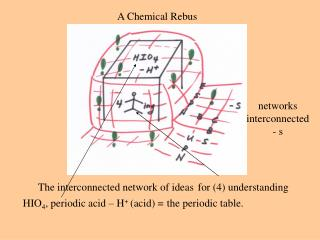DownloadDownload Presentationthe periodic table.

# the periodic table.

Télécharger la présentation## the periodic table.

- - - - - - - - - - - - - - - - - - - - - - - - - - - E N D - - - - - - - - - - - - - - - - - - - - - - - - - - -
##### Presentation Transcript

1. HIO4, periodic acid – H+ (acid) = A Chemical Rebus networks interconnected - s The interconnected network of ideas for (4) understanding the periodic table.

2. The Interconnected Network of Ideas for Understanding the Periodic Table • Periodic Law • Uniqueness Principle • Diagonal Effect • Inert Pair Effect • Metal/Non-Metal Line • Acid-Base Properties of Oxides • Standard Reduction Potentials • p-d Interactions

3. The First Five Components of the Network of Ideas Uniqueness Principle Periodic Law Diagonal Effect Metal Non-Metal Line Inert Pair Effect

4. Periodic Law Zeff inc left to right ; const vertically Radii decrease up and to the right IE, EA, EN increase up and to the right A periodic repetition of physical and chemical properties occurs when the elements are arranged in order of increasing atomic number. Figure 9.9 A Summary of the General Vertical and Horizontal Periodic Trends in Effective Nuclear Charge (Zeff), Atomic Radii (r), Ionization Energy (IE), Electron Affinities (EA), and Electronegativities (EN). This Representation of the Periodic Law Is the First Component of the Interconnected Network of Ideas for Understanding the Periodic Table.

5. Figure 9.16

6. Figure 9.6

7. Uniqueness Principle (a) The small size of the elements leading to a high degree of polarizing power and a high degree of covalent character in their compounds. (b) The greater probability of pi bonds. (c) The lack of availability of d orbitals. The chemistry of the second period elements (Li, Be, B, C, N, O, F, Ne) is quite often significantly different from that of the other elements in their respective groups. Figure 9.13 A summary of three reasons for the uniqueness principle.

8. Figure 9.5 Notice the small size of the Period 2 elements

9. The high charge density of the lithium ion polarizes the chloride ion and enhances the covalent character of the Li-Cl bond Figure 9.10 (*) Charge density: the charge-to-radius ratio of a cation or anion; taken to be directly proportional to polarizing power (the ability to produce an induced dipole) of that ion.

10. Figure 9.11 Due to the small size of the period 2 elements, π-bonds (specifically so-called pπ-pπ bonds) are more likely to form.

11. Figure 9.12

12. Figure 9.13 A summary of the three reasons for the uniqueness principle

13. Diagonal Effect A diagonal relationship exists between the chemistry of the first member of a group and that of the second member of the next group Figure 9.15 The elements of the diagonal effect. Lithium and magnesium, beryllium and aluminum, and boron and silicon, each pair diagonally located, have similar properties.

14. Fig 9.14 Four Relevant Properties of elements related to the diagonal effect

15. Inert Pair Effect The valence ns2 electronic of metallic elements, particularly those 5s2 and 6s2 pairs that follow the second- and third-row transition metals, are less reactive than we would expect on the basis of trensds in effective nuclear charge, atomic sizes, and ionization energies.

16. Metal Non-Metal Line The stepwise diagonal metal/nonmetal line divides the metals (to the bottom left of the line) from the nonmetals (to the top right of the line. The semi-metals (B, Si, Ge, As, Sb, Te, and At) are pictured along the line. Figure 9.18 The metal-nonmetal line

17. Periodic trends in Zeff, IE, EA, EN and r (b) Diagonal Effect (c) Inert Pair Effect (a) Uniqueness Principle (d) Metal-Nonmetal Line The First Five Components of the Interconnected Network of Ideas Figure 9.18, Front Left Cover & p.227

18. Nonmetals NMO =H2O=>H3O+ + NMO- Metals MO =H2O=>M+ + OH- The Sixth Component The Acid-Base Character of Oxides Figure 11.16 Front Right Cover (color) & p. 284 (B/W)

19. Eo = Standard Reduction Potential Good Reducing Agents Good Oxidizing Agents The Seventh Component Trends in Standard Reduction Potentials Figure 12.5, Back Left Cover (color) & p 318 (B/W)

20. The Eighth Component The p-d bonding involving the elements of the second and third periods Figure 15.5 Back Right Cover (color) & p 398 (B/W)